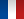• #### Number of hours

• Lectures 14.0
• Projects -
• Tutorials 14.0
• Internship -
• Laboratory works 22.0

ECTS 3.0

## Goal(s)

Provide basic notions required for the mechanical design of rotating machines for energy production.

Introduction to the theory of vibrations:

• Obtain and solve the equations of motion in simple cases and for small (linear) oscillations around equilibrium
• Understand the concepts of eigenfrequencies and eigenmodes
• Obtain eigenmodes and eigenfrequencies of discrete systems with n degrees of freedom

Responsible(s)

Barthelemy HARTHONG

## Content(s)

1- One efficient method for solving the equations of motion (20h)
Description of the motion of a mechanical system using generalised coordinates
Equations of motion (energy method)
Linearisation of the equations of motion
Free and forced oscillations of a one-degree-of-freedom system
Eigenfrequencies, eigenmodes
Vibration of systems with several degrees of freedom
2- numerical intégration of the equations of motion (8h + 22h practicals)
Deriving eigenfrequencies and eigenmodes for n-DOFs systems
Programming with python

Prerequisites

Basics of mathematics - differential equations, Taylor series, linear algebra

Test

2h exam (DS) + assignment (CC)

CC 1/3 + CT 2/3

Calendar

The course exists in the following branches:

• Curriculum - Master of Engineering GEE - Semester 5-6
see the course schedule for 2023-2024

Course language(s):